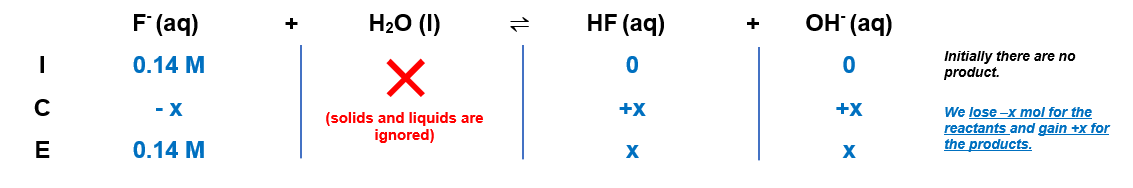# Problem: Solve an equilibrium problem (using an ICE table) to calculate the pH of each of the following solutions. (Ka(HF)=6.8 x 10-4)0.14 M NaF

###### FREE Expert SolutionCalculate Kb

$\overline{){{\mathbf{K}}}_{{\mathbf{a}}}{{\mathbf{K}}}_{{\mathbf{b}}}{\mathbf{=}}{{\mathbf{K}}}_{{\mathbf{w}}}}\phantom{\rule{0ex}{0ex}}{\mathbf{K}}_{\mathbf{b}}\mathbf{=}\frac{{\mathbf{K}}_{\mathbf{w}}}{{\mathbf{K}}_{\mathbf{a}}}\phantom{\rule{0ex}{0ex}}{\mathbf{K}}_{\mathbf{b}}\mathbf{=}\frac{\mathbf{1}\mathbf{.}\mathbf{0}\mathbf{×}{\mathbf{10}}^{\mathbf{-}\mathbf{14}}}{\mathbf{6}\mathbf{.}\mathbf{8}\mathbf{×}{\mathbf{10}}^{\mathbf{-}\mathbf{4}}}$

Kb = 1.47x10-11

96% (101 ratings)###### Problem Details

Solve an equilibrium problem (using an ICE table) to calculate the pH of each of the following solutions. (Ka(HF)=6.8 x 10-4)

0.14 M NaF